# Difference between revisions of "Connection is module structure over connection algebra"

## Statement

Let$E$ be a vector bundle over a differential manifold$M$. Then, a connection on$E$ is equivalent to giving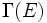$\Gamma(E)$ (the vector space of sections of$E$) the structure of a module over the connection algebra of$M$. Equivalently, it gives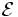$\mathcal{E}$ (the sheaf of sections of$E$) the structure of a module over the sheaf of connection algebras over$M$.

## Definitions used

### Connection

Further information: Connection

### Connection algebra

Further information: Connection algebra

## Proof

### From a connection to a module structure

The outline of the proof is as follows:

• We first show that a connection gives an action of the first-order differentiable operators on the space of sections.
• Next, we show that the Leibniz rule property of connections allows us to extend this to a well-defined action of the connection algebra.

Given: A manifold$M$, a vector bundle$E$ over$M$, a connection$\nabla$ on$E$.$B$ is the algebra of smooth fiber-preserving maps from$\Gamma(E)$ to$\Gamma(E)$.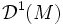$\mathcal{D}^1(M)$ is the Lie algebra of first-order differential operators on$M$ and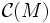$\mathcal{C}(M)$ is the connection algebra on$M$.

To prove:$\nabla$ gives rise to a homomorphism from$\mathcal{C}(M)$ to$B$.

Proof:$\nabla$ gives rise to a map: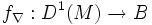$f_\nabla: D^1(M) \to B$

as follows: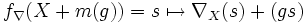$f_\nabla(X+m(g)) = s \mapsto \nabla_X(s) + (gs)$.

First observe that the map sends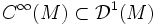$C^\infty(M) \subset \mathcal{D}^1(M)$ to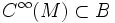$C^\infty(M) \subset B$, and is the identity restricted to that subset. In other words, the differential operator of multiplication by a function$f$, goes to the operator of multiplication by the function$f$.

We now prove some basic results about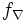$f_\nabla$:

•$f_\nabla$ is$\R$-bilinear: This is obvious.
• For any element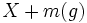$X + m(g)$ in$\mathcal{D}^1(M)$ and any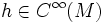$h \in C^\infty(M)$, we have$f_\nabla(m(g) \dot (X + m(h))(s) = m(g)f_\nabla(X + m(h))(s)$. This essentially follows from the fact that a connection is tensorial in the direction of differentiation: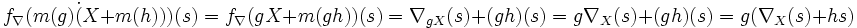$f_\nabla(m(g) \dot (X + m(h)))(s) = f_\nabla(gX + m(gh))(s) = \nabla_{gX}(s) + (gh)(s)= g\nabla_X(s) + (gh)(s) = g(\nabla_X(s) + hs)$.

• For any element$X + m(g)$ in$\mathcal{D}^1(M)$ and any$h \in C^\infty(M)$, we have$f_\nabla((X + m(h)) \dot m(g))(s) = f_\nabla(X + m(h))(m(g)s)$. This essentially follows from the Leibniz rule property.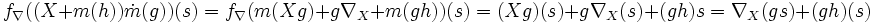$f_\nabla((X + m(h)) \dot m(g))(s) = f_\nabla(m(Xg) +g\nabla_X + m(gh))(s) = (Xg)(s) + g\nabla_X(s) + (gh)s = \nabla_X(gs) + (gh)(s)$.